# 1.本章学习总结

## 1.1 学习内容总结

• 结构体struct是类似于一个数组的元素 但其中可以存放多个不同种类的变量
• 结构体的定义：如
typedef struct you{
int number;
char name;
int  score;
struct you *next;
}YOU;


• 结构体的嵌套定义 可在结构体里用另一个结构体
• 结构体的递归 可在结构体里定义一个结构指针指向它本身 用于键链表
• 结构体的初始化：可用花括号 如：
struct stu s1={101,"zhang",67,78,67};


• 可用sizeof(结构体变量）来计算其所占内存空间。

• 结构体成员的引用：结构体变量名+.+结构体成员
例如s1.num

• 结构体变量的整体赋值 可直接s2=s1 相当于把每个成员都赋值。

• 结构体变量作为函数参数 通常传指针

• 结构体指针用法：struct stu* p 若想引用结构体成员可用->表示 如

p->num等同于*p.num

• 枚举
enum 枚举名{枚举值1，枚举值2，...} 变量列表；

• fgetc
从文件中逐个读入字符到char
读到文件末尾返回eof
ch=fgetc(fp)

• fputc
将一个字符ch写到文件中
fputc(ch,fp)

## 简易的复制文件代码用fgets fputs

#include <stdio.h>
#include<stdlib.h>
int main()
{
char ch;
FILE *fp1,*fp2;
{
printf("kong");
exit(0);
}
{
printf("kong");
exit(0);
}
while(!feof(fp1))
{

ch=fgetc(fp1);
**if(ch!=EOF)**
fputc(ch,fp2);
//用fgetc从文件1读入字符到ch中再用fputc将ch写入文件2
}
if(fclose(fp1))
{
printf("can't close the file");
exit(0);
}
if(fclose(fp1))
{
printf("can't close the file");
exit(0);
}

}


• fgets(array, number,fp)
将数量为number的值读入到array中

• fputs(s,fp)
写入一个字符串到文件中

• feof

while(!feof(fp1))


• fclose

 if(fclose(fp))
{
printf("can't close the file");
exit(0);
}

• rewind(FILE *fp)
将文件指针摆正

• fseek(fp,offset,from)
offset是long型数据 使用常量时 后缀加L
from：从哪里开始偏移
0:文件首
1:当前位置
2:文件尾部
example
fseek(fp,-10L,2)

• ftell(fp)
获取当前指针位置相对于头文件位置的偏移量

# 2.综合作业--“我爱成语”

### 2.1 文件介绍

1.头文件介绍

• struct LOG 用于用户登录

• struct IDI 用于存储成语数据

• struct RANK 用于存储以及修改排名数组

• Checkuser() 用于用户登录

• Getdata() 用于获取成语数据

• Getquestion()用于出题

• Rerank()用于重新排名并写入文件

• Sortlist()用来给成绩排序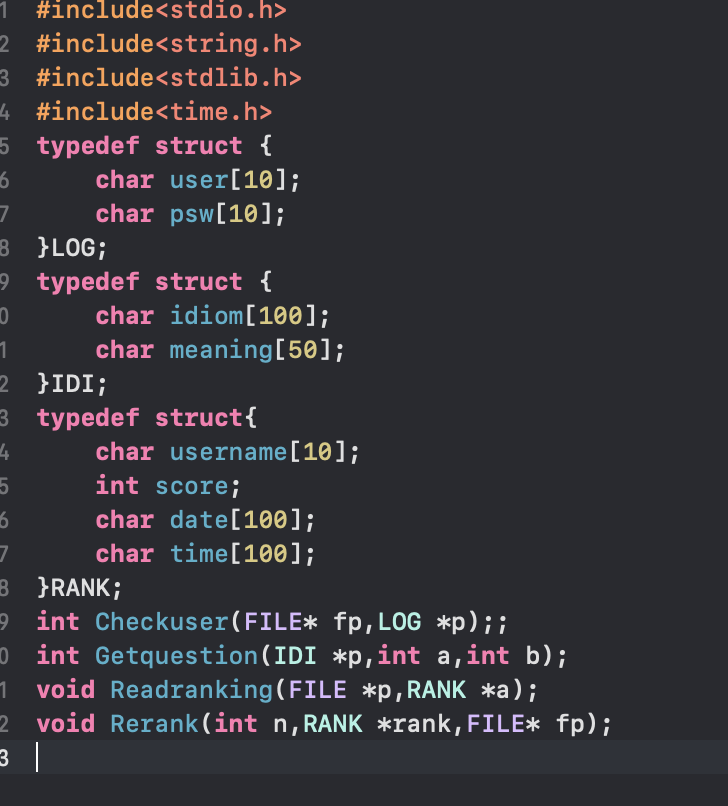### 2.函数实现文件介绍

• 在主函数中作为登录系统 先在函数外打开文件 并将文件指针fp和结构指针p传入函数 来检测密码

•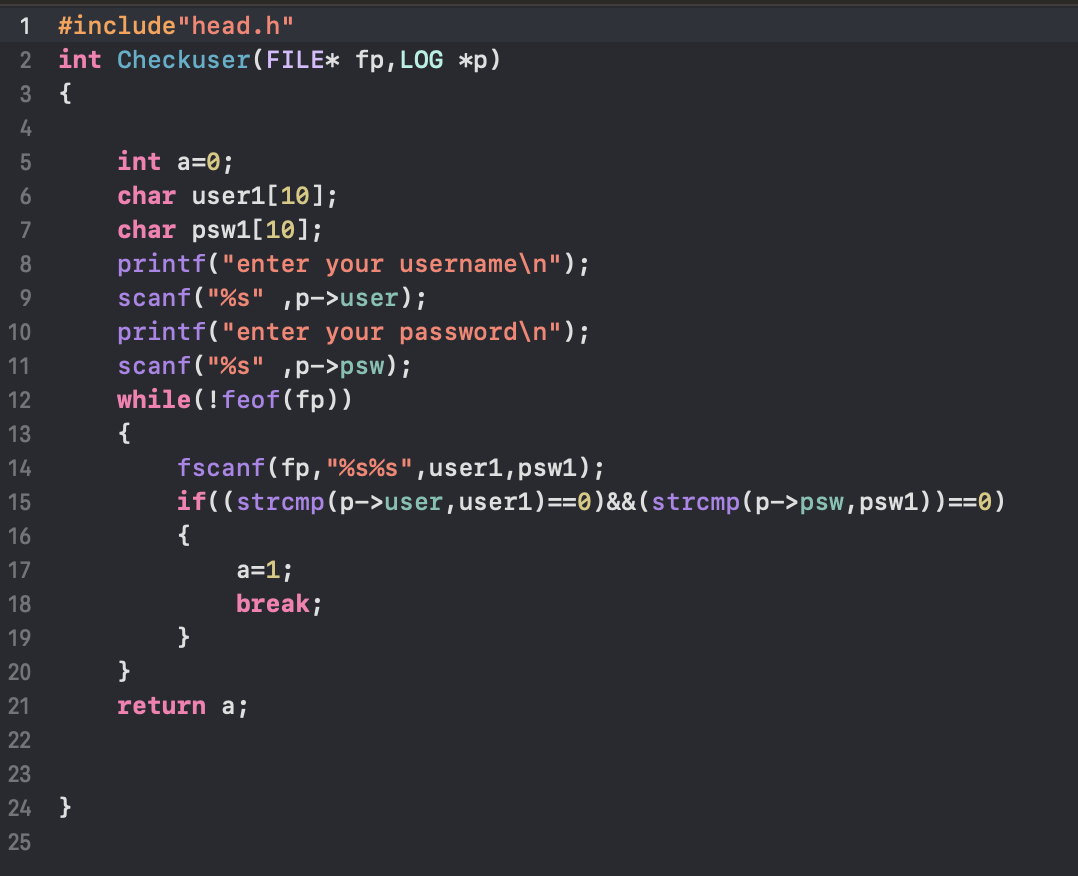文件2:Getdata.c

• 将文件中的成语全部读入IDI array这个结构体数组中

•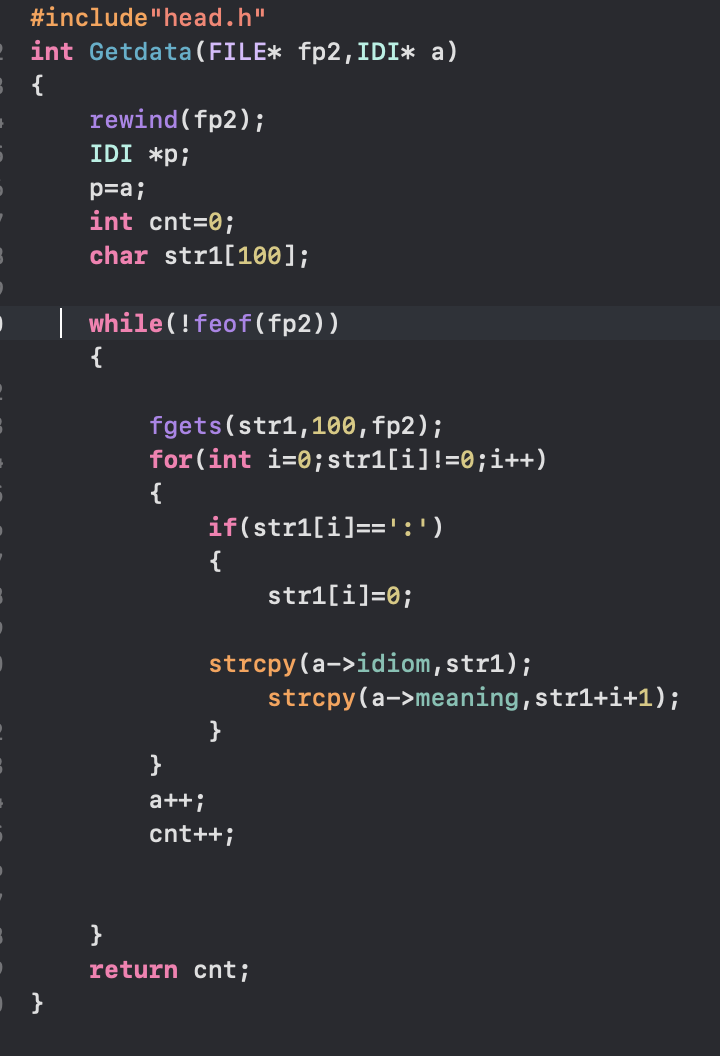文件3:Getquestion.c
用来出题 将Getdata函数读到的数据随机挖空出题

#include"head.h"
int Getquestion(IDI* p, int a, int b, int score)

{

char str;

switch (a)
{
case 1:
printf("%c%c", p[b].idiom, p[b].idiom);
printf("%c%c__\n", p[b].idiom, p[b].idiom);
break;
case 2:
printf("%c%c_", p[b].idiom, p[b].idiom);
printf("%c%c_\n", p[b].idiom, p[b].idiom);
break;
case 3:
printf("__%c%c", p[b].idiom, p[b].idiom);
printf("%c%c\n", p[b].idiom, p[b].idiom);
break;
case 4:
printf("_%c%c", p[b].idiom, p[b].idiom);
printf("%c%c_\n", p[b].idiom, p[b].idiom);
break;

}
scanf("%s", str);

if (strcmp(str, p[b].idiom) == 0)
{
score += 10;
}
else {
score = score - 4;
}

return score;

}


• 用于读入排行数据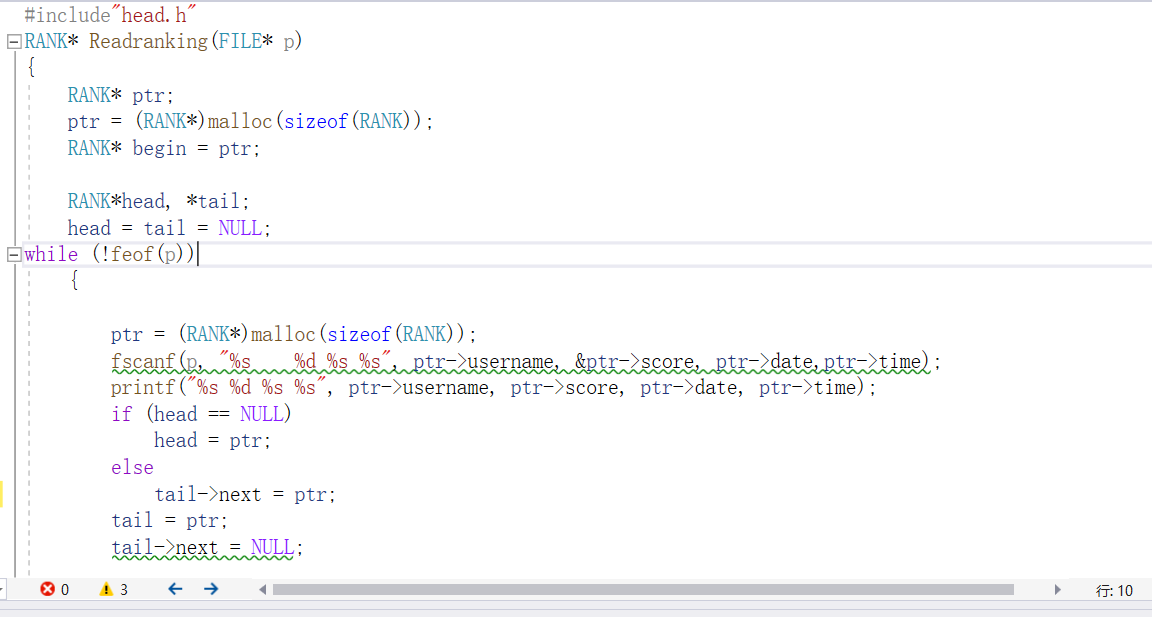文件4:Reranking.c
用来更新排行数据并存入文件中
其中调用一次Sortlist函数 再把传入的文件指针关闭 重新打开时用w模式覆盖掉原来的文件 再用链表的遍历加fprint函数将排好名字的数据重新写入文件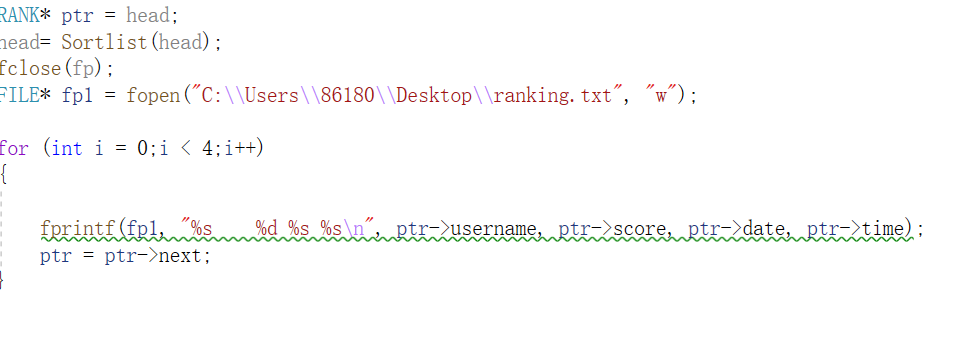文件4:Sortlist.c
用于给用户更新的数据排名
用链表的选择排序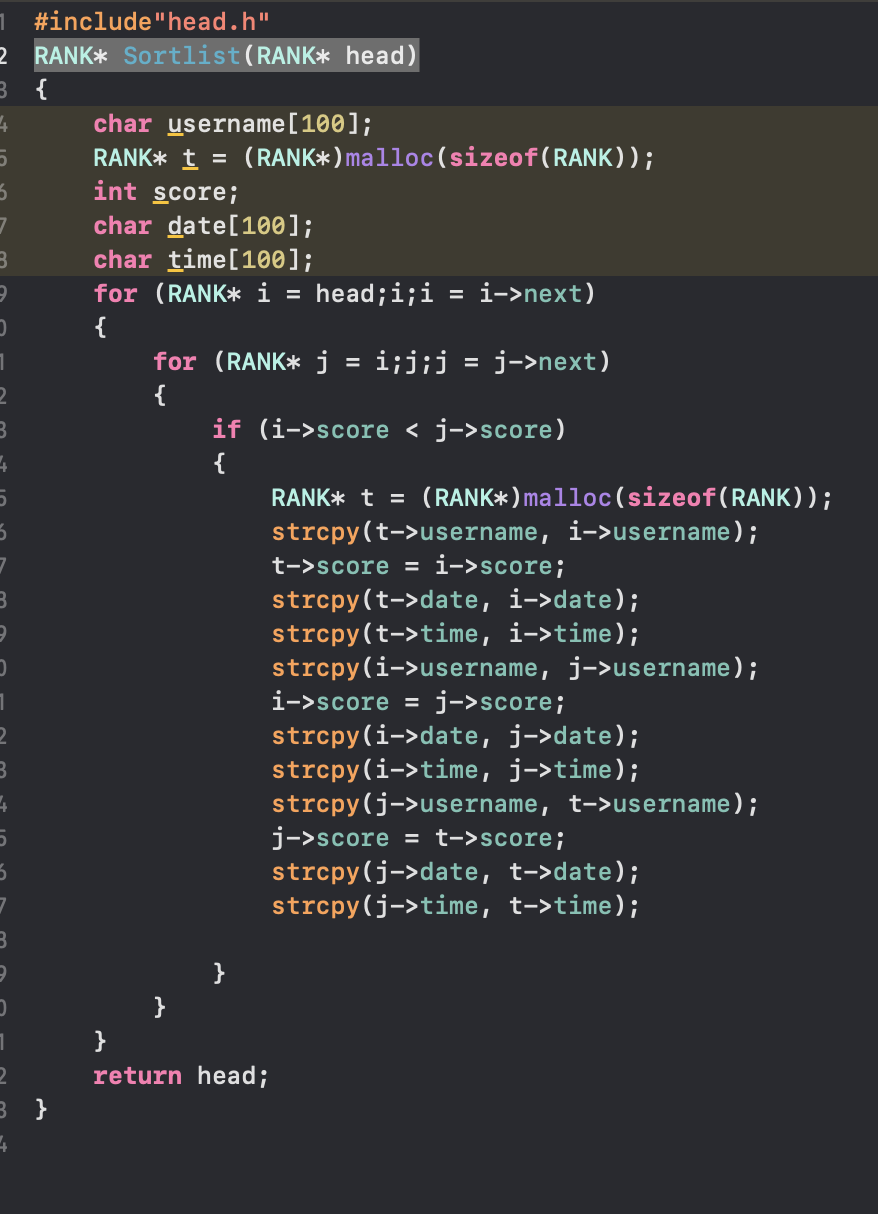## 2.2 运行结果

• 菜单界面
• 这边输出了保存到结构体中的数据 确保读入无误。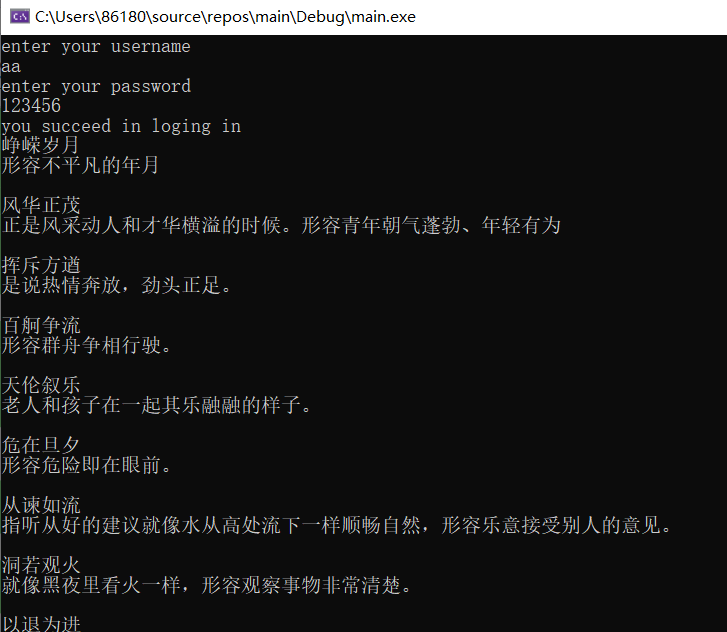出题界面
答对一题得10分 答错一题扣4 分 一共十题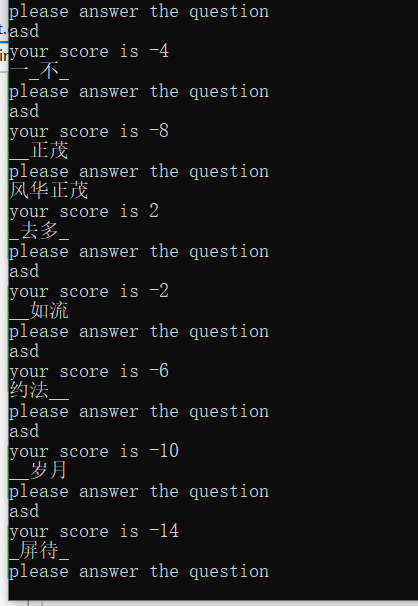• 最后排序前和排序后的界面
•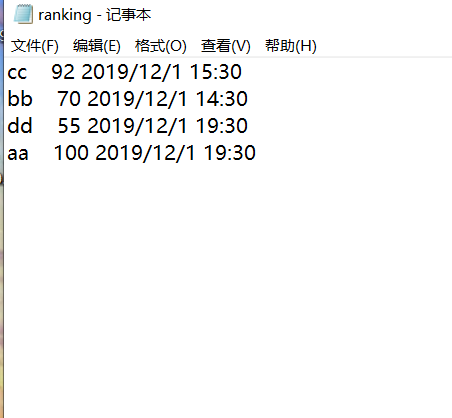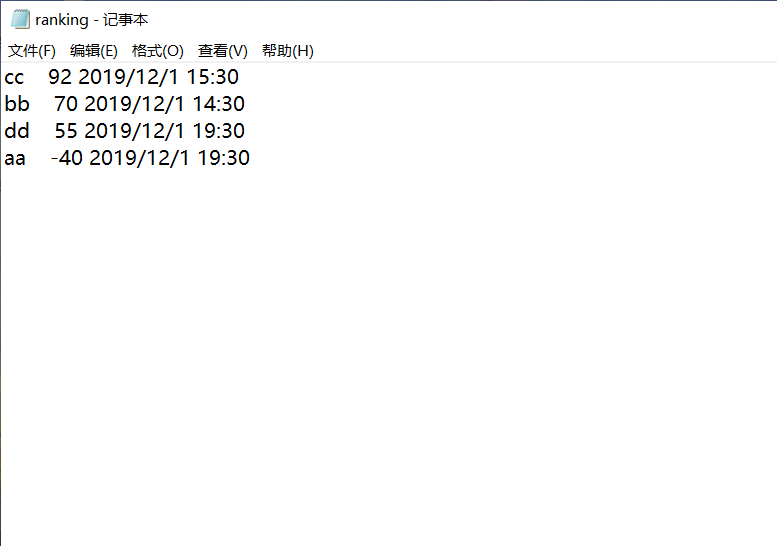• 其中我先让aa用户全做错 后让dd用户答对一题 答错九题 可看出排序成功

# 2.3 大作业总结

## 1.碰到的问题及解决方法

• 建链表时忘记用malloc函数 导致崩溃
• 犯了一个很简单的错误int score=0; int score=Getdata() 定义了两次score 导致程序崩溃